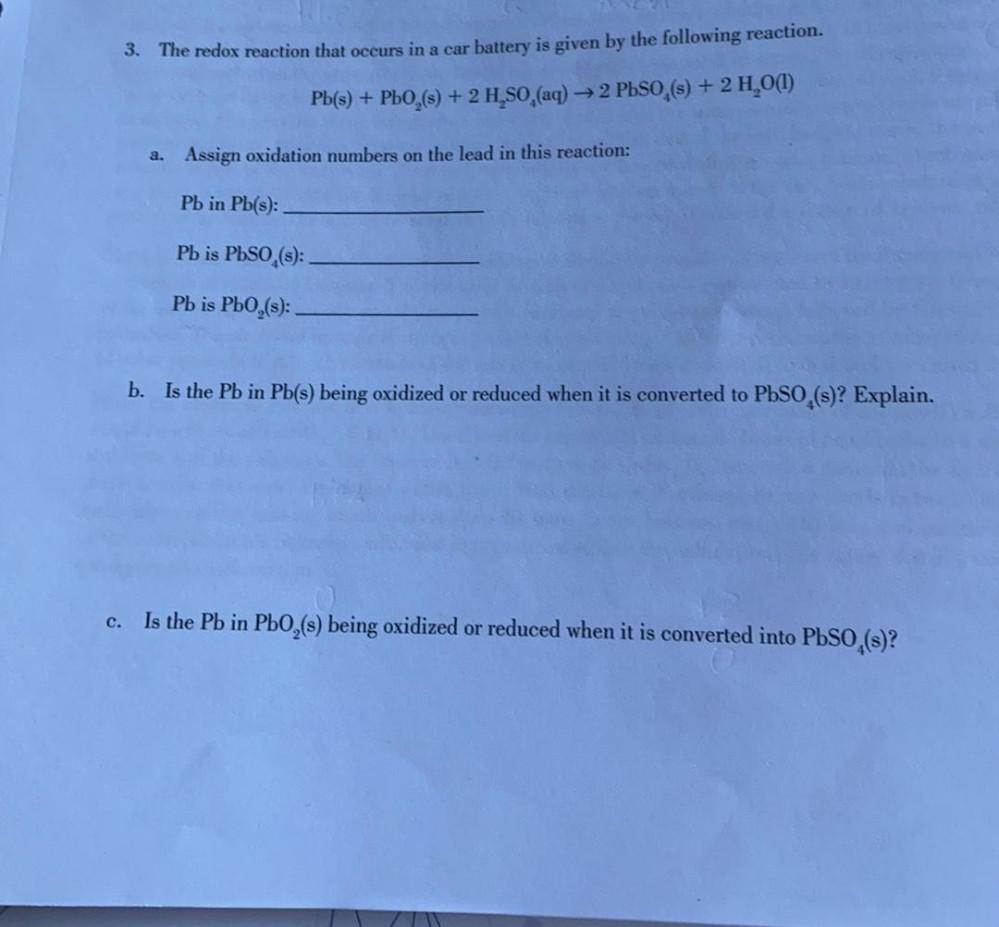Question:

# 3. The redox reaction that occurs in a car battery is given by the following reaction. Pb(s) + PLO,(s) + 2 H,80,(aq) → 2 PbSO,(s3. The redox reaction that occurs in a car battery is given by the following reaction. Pb(s) + PLO,(s) + 2 H,80,(aq) → 2 PbSO,(s) + 2 H,0(1) + → 2 a. Assign oxidation numbers on the lead in this reaction: Pb in Pb(s): Pb is PbSO,(s): Pb is PbO,(s): b. Is the Pb in Pb(s) being oxidized or reduced when it is converted to PbSO,(s)? Explain. C. Is the Pb in PbO,(s) being oxidized or reduced when it is converted into PbSO,(s)?